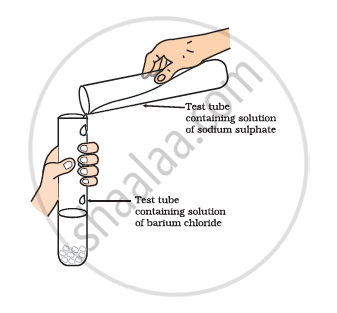# Give an Example of a Double Displacement Reaction Other than the One Given in Activity. - Science

Give an example of a double displacement reaction other than the one given in the following Activity.
•Take about 3 mL of sodium sulphate
solution in a test tube.
• In another test tube, take about 3 mL of
barium chloride solution.
• Mix the two solutions given in the following fig.
• What do you observe?#### Solution

2KBr (aq) + BaI2 (aq) → 2KI (aq) + BaBr2 (aq)

Concept: Types of Chemical Change or Chemical Reaction - Double Displacement Reaction
Is there an error in this question or solution?
Chapter 1: Chemical Reactions and Equations - Intext Questions [Page 13]

#### APPEARS IN

NCERT Class 10 Science
Chapter 1 Chemical Reactions and Equations
Intext Questions | Q 2 | Page 13

Share